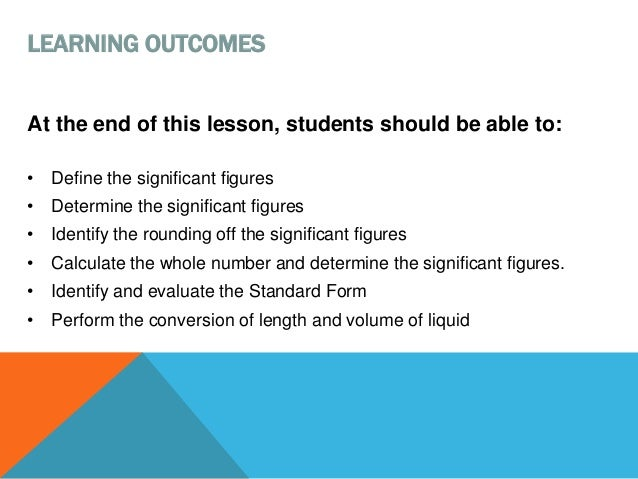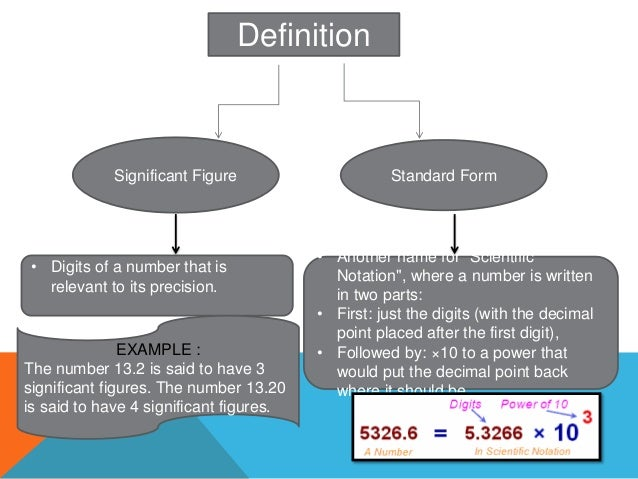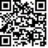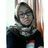Successfully reported this slideshow.Upcoming SlideShare
×

ofUpcoming SlideShare
F4 01 Standard Form
Next

20 Likes

Share

# Chapter 1 : SIGNIFICANT FIGURES AND STANDARD FORM

Define the significant figures
Determine the significant figures
Identify the rounding off the significant figures
Calculate the whole number and determine the significant figures.
Identify and evaluate the Standard Form
Perform the conversion of length and volume of liquid .

See all

See all

### Chapter 1 : SIGNIFICANT FIGURES AND STANDARD FORM

1. 1. TOPIC 1 SIGNIFICANT FIGURES AND STANDARD FORM
2. 2. At the end of this lesson, students should be able to: • Define the significant figures • Determine the significant figures • Identify the rounding off the significant figures • Calculate the whole number and determine the significant figures. • Identify and evaluate the Standard Form • Perform the conversion of length and volume of liquid LEARNING OUTCOMES
3. 3. Definition Significant Figure Standard Form • Digits of a number that is relevant to its precision. EXAMPLE : The number 13.2 is said to have 3 significant figures. The number 13.20 is said to have 4 significant figures. • Another name for "Scientific Notation", where a number is written in two parts: • First: just the digits (with the decimal point placed after the first digit), • Followed by: ×10 to a power that would put the decimal point back where it should be.
4. 4. Significant Figures Determination Of Significant Figures Rounding Off Significant Figures Non-Zero Digits Zero occurring between non- zero digits In Decimal Rules
5. 5. Non-Zero Digits All non-zero digits are significant: 312 : 3 significant figures 3149 : 4 significant figures 283.847 : 6 significant figures
6. 6. Zero Occurring Between Non-Zero Digits Zeros occurring between non-zero digits are significant: 6003 : 4 significant figures 201 : 3 significant figures 98908 : 5 significant figures
7. 7. In Decimal In a decimal, zeros after the non-zero digits are significant: 8.00 : 3 significant figures 99.0000 : 6 significant figures 10.00 : 4 significant figures In a decimal, all zeros before a non-zero digit are not significant: 0.009 : 1 significant figures 0.01 : 1 significant figures 0.10 : 2 significant figures
8. 8. EXERCISES
9. 9. Rounding Off Significant Figures Rules CASE A: In rounding off numbers, the last figure kept should be unchanged if the first figure dropped is less than 5. EXAMPLE : If only one decimal is to be kept, then 6.422 becomes 6.4. CASE C: In rounding off numbers, the last figure kept should be increased by 1 if the first figure dropped is greater than 5. EXAMPLE : If only two decimals are to be kept, then 6.4872 becomes 6.49. Similarly, 6.997 becomes 7.00. CASE B: In rounding off numbers, if the first figure dropped is 5, and all the figures following the five are zero or if there are no figures after the 5, then the last figure kept should be increased by 1 if that last figure is odd. EXAMPLE : For example, if only two decimals are to be kept, then 6.755000 becomes 6.76.
10. 10. Standard Form: Converting single number to Standard Form
11. 11. Standard Form: Converting numbers in Standard Form to Single Numbers
12. 12. Conversion
13. 13. THE END Please Do a Lot of Exercises THANK YOU JUST REMEMBER:
•#### MichelleThomas104508

Dec. 4, 2021
•#### HibaFatima18

Sep. 26, 2020
•#### FazleYousafzai

May. 21, 2020
•#### AnnePenelopeGutierrez

Mar. 26, 2020
•Dec. 17, 2019
•#### amyryuzaini

Dec. 17, 2018
•#### HaNn4

Jan. 25, 2018
•#### keluargaku1985

Jan. 8, 2018
•#### rohanamuhdrejab

Jan. 8, 2018
•#### AnnieLoo3

Jan. 4, 2018
•#### StevenNkata

Jan. 1, 2018
•#### NehaGautam8

Jul. 26, 2017
•#### RoshiniHarry

Jul. 10, 2017
•#### NajwaAbdullah5

May. 4, 2017
•#### cikwani2

Feb. 17, 2017
•#### roziey77

Jan. 7, 2017
•#### ssusere1fa52

Dec. 24, 2016
•#### CeciliaBo1

Mar. 19, 2016
•Jan. 11, 2016
•#### ijazatiaqmar

Dec. 20, 2014

Total views

48,346

On Slideshare

0

From embeds

0

Number of embeds

261

564

Shares

0